### Home > CALC > Chapter 6 > Lesson 6.1.3 > Problem6-34

6-34.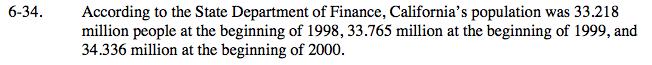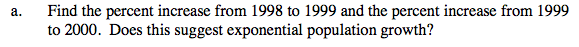Calculate this value with the two different data sets. Are they nearly the same?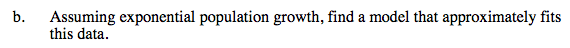P(t) = P0(1 − rate)t
Let the year 1998 correspond with t = 0.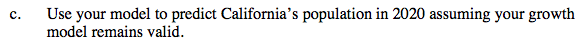Evaluate P(22).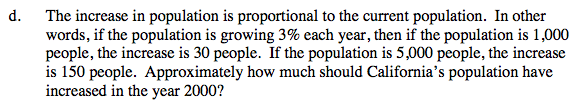Answers will vary depending on the rate estimate. ≈ 34,336,000(rate)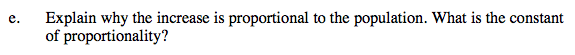Approximate growth in population = P0(growth factor).
Find growth factor in part (a).